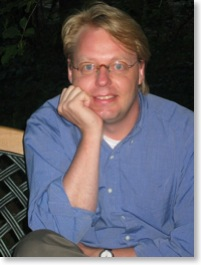# Nodal Discontinuous Galerkin Methods for solving Partial Differential Equations

## Ph.D. Course on Nodal Discontinuous Galerkin Methods for solving Partial Differential EquationsWe offer a Ph.D. course introducing advanced Discontinuous Galerkin Methods for solving Partial Differential Equations.

The course is offered with support from the DTU Informatics Graduate School (ITMAN) and the Danish Center for Applied Mathematics and Mechanics (DCAMM) at Technical University of Denmark and is a complementary scientific computing activity of GPUlab.

The aim of the course is to give the students an introduction to discontinuous Galerkin methods (DG-FEM) for solving problems in the engineering and the sciences described by systems of partial differential equations. These methods, most appropriately considered as a combination of finite volume and finite element methods, have become widely used during the last decade as a powerful tool for the simulation of challenging problems in the sciences and engineering.

The PhD course covers both an overview of the theoretical properties of the methods, their efficient implementation, and more applied problems related to the multi-dimensional problems, unstructured grids and grid-generation, illustrated using Matlab. We shall draw on application examples and illustrations from electromagnetics, fluid and gas dynamics but the focus on the course is on understanding the methods in sufficient depth to apply them to a broad range of problems.

## Learning objectives:

A student who has met the objectives of the course will be able to:

• Apply the basic ideas underlying discontinuous Galerkin methods.
• Apply how the DG-FEM methods are used as building blocks in the simulation of phenomena descibed by partial differential equations.
• Identify and exploit the properties and structutre of the underlying problem.
• Be able to complete basic analysis to formulate a suitable scheme for a new problem.
• Implement such methods and extensions in Matlab using the provided Matlab based toolbox.
• Skillfully perform numerical experiments.
• Analyse and explain the observed behavior of the methods based on a basic theoretical insight.
• Apply important principles underlying the use of modern numerical methods in selected applications.

## Course description

The full course description can be found here [LINK].

## Responsible:

The course will be given by:

Richard Petersens Plads, DTU - Bygning 321, DK-2800 Lyngby
k.haugland@mat.dtu.dk